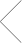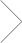# Trigonometry Tutoring in Des Moines, Iowa.

In Home & Online Trigonometry Tutoring with a Club Z! Tutor.

# Get Tutoring Info Now

## Our office provides in home and online trigonometry services in the following locations including Des Moines, Clive, Waukee, Urbandale, and Johnston in Iowa.

### Trigonometry Tutoring: In Home & Online

The mathematical subject of trigonometry has tremendous applications in the sciences, engineering and technology. It’s also even used in video games! Our Club Z! trigonometry tutors help your student succeed, and become a leader in the classroom. Our trigonometry tutors will develop a personal program for your student and will help them understand the basics, or complex trigonometric functions.

Club Z! Tutoring of Des Moines, IA Trigonometry Tutors can help your student in all of the following areas of Trigonometry:

1. Oblique triangles
2. Functions
3. Right triangle trigonometry and basic identities
4. Trigonometric identities
5. Inverse trigonometric
6. Inverse trigonometric
7. Logarithmic functions
8. and Much More!

Club Z! Tutoring has been helping students for the past 20 years understand Pythagoreans method, and other forms of trigonometry. Currently we have 400 offices across the United States, and thousands of in home and online tutors that are ready to help you learn all forms of trigonometry.

In addition to Des Moines, our office offers trigonometry tutoring services in the following locations: Clive, Waukee, Urbandale, and Johnston in Iowa.

If you’re interested in finding a custom-tailored trigonometry tutoring program in your area please fill out the form above, or call 515-225-1909.

#### Subjects We TutorElementary Math

Elementary Math Tutoring with a Club Z! Tutor. Club Z! Tutoring of Des Moines, has highly qualified and experienced elementary math tutors who provide one-on-one, focused instruction for all elementary math topics, including addition and subtraction, multiplication, division, fractions, and even Common Core math. Our elementary math tutors will work with your student to not only become proficient, but also a leader in the classroom.Trigonometry

Trigonometry Tutoring with a Club Z! Tutor. Club Z! Tutoring of Des Moines, trigonometry tutors help your student succeed. Our Trigonometry tutors develop personal programs that assess your students trigonometry needs. Our trigonometry Tutors can help your student in all of the following areas of Trigonometry: Oblique triangles, Functions, Right triangle trigonometry and basic identities, Trigonometric identities, Inverse trigonometric, Inverse trigonometric and much more.Calculus

Calculus Tutoring with a Club Z! Tutor. Club Z! Tutoring of Des Moines, provides you with calculus tutors you can count on. Our Club Z! calculus tutors have a minimum of a bachelors degree, and have years of calculus tutoring experience. Whether it is graphing linear functions or solving differential equations, our calculus tutors are able to help you to excel at every level, including: Pre-Calculus, High School Calculus, AP Calculus, Honors Calculus, and College Level Calculus.Geometry

Geometry Tutoring with a Club Z! Tutor. Club Z! Tutoring of Des Moines, geometry tutor develops a unique geometry tutoring program and lesson plan with the student. Our geometry tutors are able to help you excel at every level, including: High School Geometry, Honors Geometry, Middle School Geometry, and College Level Geometry. Our geometry tutors will focus on teaching your student the bestAlgebra

Algebra Tutoring with a Club Z! Tutor. Club Z! Tutoring of Des Moines, algebra tutor develops a unique algebra tutoring program and lesson plan, focused on helping students succeed in algebra. Our algebra tutors prepare their students for algebra tests and quizzes, assist them with algebra homework, help improve classroom performance, and increase their understanding and confidence level in algebra.

Find the right fit or it’s free.

We guarantee you’ll find the right tutor, or we’ll cover the first hour of your lesson.